# select count(1) 和select count(*)的区别

select count(1) 和select count(*)都是统计某个表中或者视图的记录条数，那他们两个有什么区别呢？以下我将做下试验。

go
--在运行前开启时间和IO统计的设置，选中显示执行计划
--set statistics time on
--set statistics io on

select COUNT(*) from dbo.DatabaseLog

SQL Server parse and compile time:
CPU time = 0 ms, elapsed time = 0 ms.

SQL Server Execution Times:
CPU time = 0 ms,  elapsed time = 0 ms.
SQL Server parse and compile time:
CPU time = 0 ms, elapsed time = 0 ms.

SQL Server Execution Times:
CPU time = 0 ms,  elapsed time = 0 ms.
SQL Server parse and compile time:
CPU time = 0 ms, elapsed time = 19 ms.

(1 row(s) affected)

(1 row(s) affected)

SQL Server Execution Times:
CPU time = 0 ms,  elapsed time = 78 ms.
SQL Server parse and compile time:
CPU time = 0 ms, elapsed time = 0 ms.

SQL Server Execution Times:
CPU time = 0 ms,  elapsed time = 0 ms.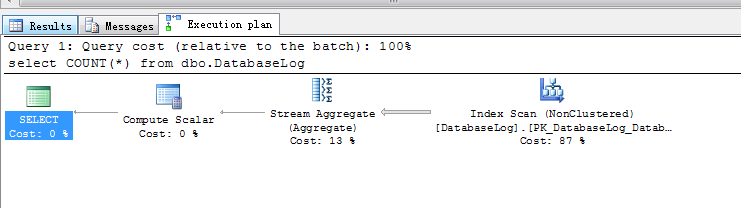SQL Server parse and compile time:
CPU time = 0 ms, elapsed time = 0 ms.

SQL Server Execution Times:
CPU time = 0 ms,  elapsed time = 0 ms.
SQL Server parse and compile time:
CPU time = 0 ms, elapsed time = 0 ms.

SQL Server Execution Times:
CPU time = 0 ms,  elapsed time = 0 ms.
SQL Server parse and compile time:
CPU time = 0 ms, elapsed time = 1 ms.

(1 row(s) affected)

(1 row(s) affected)

SQL Server Execution Times:
CPU time = 0 ms,  elapsed time = 1 ms.
SQL Server parse and compile time:
CPU time = 0 ms, elapsed time = 0 ms.

SQL Server Execution Times:
CPU time = 0 ms,  elapsed time = 0 ms.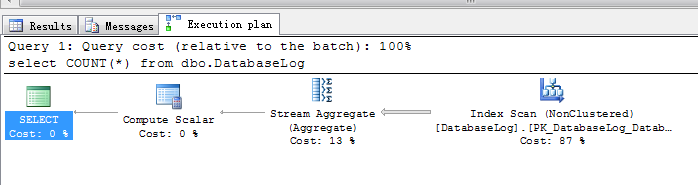go
--dbcc freeproccache
--set statistics time on
--set statistics io on

select COUNT(1) from dbo.DatabaseLog

SQL Server parse and compile time:
CPU time = 0 ms, elapsed time = 0 ms.

SQL Server Execution Times:
CPU time = 0 ms,  elapsed time = 0 ms.
SQL Server parse and compile time:
CPU time = 0 ms, elapsed time = 0 ms.

SQL Server Execution Times:
CPU time = 0 ms,  elapsed time = 0 ms.
SQL Server parse and compile time:
CPU time = 0 ms, elapsed time = 1 ms.

(1 row(s) affected)

(1 row(s) affected)

SQL Server Execution Times:
CPU time = 0 ms,  elapsed time = 1 ms.
SQL Server parse and compile time:
CPU time = 0 ms, elapsed time = 0 ms.

SQL Server Execution Times:
CPU time = 0 ms,  elapsed time = 0 ms.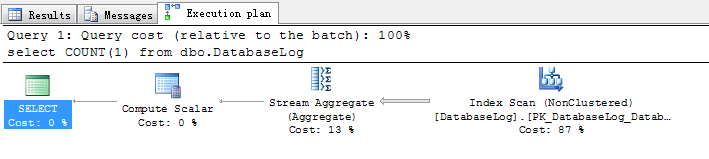SQL Server parse and compile time:
CPU time = 0 ms, elapsed time = 0 ms.

SQL Server Execution Times:
CPU time = 0 ms,  elapsed time = 0 ms.
SQL Server parse and compile time:
CPU time = 0 ms, elapsed time = 0 ms.

SQL Server Execution Times:
CPU time = 0 ms,  elapsed time = 0 ms.
SQL Server parse and compile time:
CPU time = 0 ms, elapsed time = 0 ms.

(1 row(s) affected)

(1 row(s) affected)

SQL Server Execution Times:
CPU time = 0 ms,  elapsed time = 1 ms.
SQL Server parse and compile time:
CPU time = 0 ms, elapsed time = 0 ms.

SQL Server Execution Times:
CPU time = 0 ms,  elapsed time = 0 ms.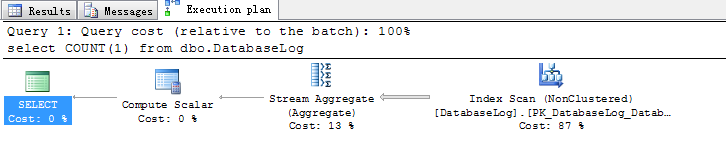go
--set statistics time on
--set statistics io on

SQL Server parse and compile time:
CPU time = 0 ms, elapsed time = 0 ms.

SQL Server Execution Times:
CPU time = 0 ms,  elapsed time = 0 ms.
SQL Server parse and compile time:
CPU time = 0 ms, elapsed time = 0 ms.

SQL Server Execution Times:
CPU time = 0 ms,  elapsed time = 0 ms.
SQL Server parse and compile time:
CPU time = 0 ms, elapsed time = 1 ms.

(1 row(s) affected)

(1 row(s) affected)

SQL Server Execution Times:
CPU time = 0 ms,  elapsed time = 92 ms.
SQL Server parse and compile time:
CPU time = 0 ms, elapsed time = 0 ms.

SQL Server Execution Times:
CPU time = 0 ms,  elapsed time = 0 ms.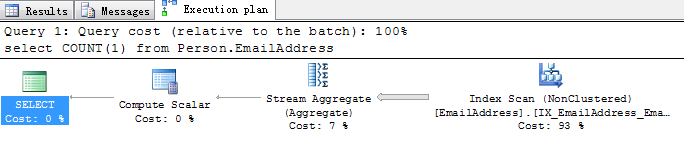SQL Server parse and compile time:
CPU time = 0 ms, elapsed time = 0 ms.

SQL Server Execution Times:
CPU time = 0 ms,  elapsed time = 0 ms.
SQL Server parse and compile time:
CPU time = 0 ms, elapsed time = 0 ms.

SQL Server Execution Times:
CPU time = 0 ms,  elapsed time = 0 ms.
SQL Server parse and compile time:
CPU time = 0 ms, elapsed time = 0 ms.

(1 row(s) affected)

(1 row(s) affected)

SQL Server Execution Times:
CPU time = 0 ms,  elapsed time = 5 ms.
SQL Server parse and compile time:
CPU time = 0 ms, elapsed time = 0 ms.

SQL Server Execution Times:
CPU time = 0 ms,  elapsed time = 0 ms.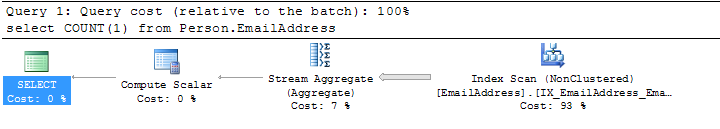dbcc freeproccache

go
--set statistics time on
--set statistics io on

SQL Server parse and compile time:
CPU time = 0 ms, elapsed time = 0 ms.

SQL Server Execution Times:
CPU time = 0 ms,  elapsed time = 0 ms.
SQL Server parse and compile time:
CPU time = 0 ms, elapsed time = 0 ms.

SQL Server Execution Times:
CPU time = 0 ms,  elapsed time = 0 ms.
SQL Server parse and compile time:
CPU time = 0 ms, elapsed time = 0 ms.

(1 row(s) affected)

(1 row(s) affected)

SQL Server Execution Times:
CPU time = 0 ms,  elapsed time = 5 ms.
SQL Server parse and compile time:
CPU time = 0 ms, elapsed time = 0 ms.

SQL Server Execution Times:
CPU time = 0 ms,  elapsed time = 0 ms.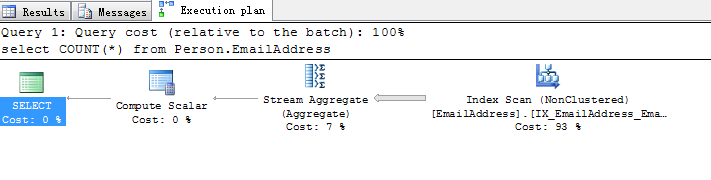SQL Server parse and compile time:
CPU time = 0 ms, elapsed time = 0 ms.

SQL Server Execution Times:
CPU time = 0 ms,  elapsed time = 0 ms.
SQL Server parse and compile time:
CPU time = 0 ms, elapsed time = 0 ms.

SQL Server Execution Times:
CPU time = 0 ms,  elapsed time = 0 ms.
SQL Server parse and compile time:
CPU time = 0 ms, elapsed time = 0 ms.

(1 row(s) affected)

(1 row(s) affected)

SQL Server Execution Times:
CPU time = 0 ms,  elapsed time = 5 ms.
SQL Server parse and compile time:
CPU time = 0 ms, elapsed time = 0 ms.

SQL Server Execution Times:
CPU time = 0 ms,  elapsed time = 0 ms.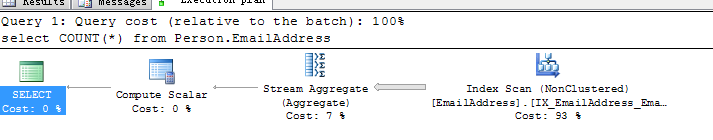CREATE TABLE TEST_COUNT
(
ID  INT NOT NULL,
COL1 VARCHAR(10) NULL,
COL2 VARCHAR(13) NULL,
COL3 DATETIME NULL,
COL4 DATETIME NULL
)

DECLARE @A INT
SELECT @A=0
WHILE (@A<100000)
BEGIN
INSERT INTO TEST_COUNT
SELECT @A,CAST(REPLICATE(@A,10) AS VARCHAR(10)),CAST(REPLICATE(@A,10) AS VARCHAR(10)),GETDATE(),GETDATE()
SELECT @A=@A+1
END

USE TEST
GO
--dbcc freeproccache
select COUNT(*) from dbo.TEST_COUNT

SQL Server parse and compile time:
CPU time = 0 ms, elapsed time = 0 ms.

SQL Server Execution Times:
CPU time = 0 ms,  elapsed time = 0 ms.
SQL Server parse and compile time:
CPU time = 0 ms, elapsed time = 0 ms.

SQL Server Execution Times:
CPU time = 0 ms,  elapsed time = 0 ms.
SQL Server parse and compile time:
CPU time = 0 ms, elapsed time = 0 ms.

(1 row(s) affected)

(1 row(s) affected)

SQL Server Execution Times:
CPU time = 16 ms,  elapsed time = 20 ms.
SQL Server parse and compile time:
CPU time = 0 ms, elapsed time = 0 ms.

SQL Server Execution Times:
CPU time = 0 ms,  elapsed time = 0 ms.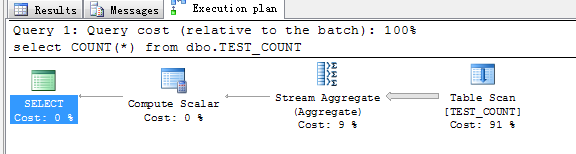USE TEST
GO
--dbcc freeproccache
select COUNT(1) from dbo.TEST_COUNT

SQL Server parse and compile time:
CPU time = 0 ms, elapsed time = 0 ms.

SQL Server Execution Times:
CPU time = 0 ms,  elapsed time = 0 ms.
SQL Server parse and compile time:
CPU time = 0 ms, elapsed time = 0 ms.

SQL Server Execution Times:
CPU time = 0 ms,  elapsed time = 0 ms.
SQL Server parse and compile time:
CPU time = 0 ms, elapsed time = 1 ms.

(1 row(s) affected)

(1 row(s) affected)

SQL Server Execution Times:
CPU time = 15 ms,  elapsed time = 23 ms.
SQL Server parse and compile time:
CPU time = 0 ms, elapsed time = 0 ms.

SQL Server Execution Times:
CPU time = 0 ms,  elapsed time = 0 ms.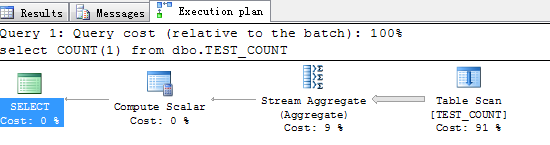CREATE TABLE DBO.TEST_COUNT
(
ID  INT NOT NULL,
COL1 VARCHAR(10) NULL,
COL2 VARCHAR(13) NULL,
COL3 DATETIME NULL,
COL4 DATETIME NULL,
col5 int null,
col6 bit null
)
DECLARE @A INT
SELECT @A=0
WHILE (@A<100000)
BEGIN
INSERT INTO TEST_COUNT
SELECT @A,CAST(REPLICATE(@A,10) AS VARCHAR(10)),CAST(REPLICATE(@A,10) AS VARCHAR(10)),GETDATE(),GETDATE(),@A,null
SELECT @A=@A+1
END
alter  table  DBO.TEST_COUNT
add  constraint PK_TEST_COUNT primary key (ID ASC)
CREATE NONCLUSTERED INDEX IX_TEST_COUNT_COL4 ON DBO.TEST_COUNT
(
COL4
)

CREATE NONCLUSTERED INDEX IX_TEST_COUNT_col55 ON DBO.TEST_COUNT
(
col5
)WHERE (COL5>100)

CREATE NONCLUSTERED INDEX IX_TEST_COUNT_col6 ON DBO.TEST_COUNT
(
col6
)
SELECT COUNT(*) FROM dbo.TEST_COUNT         --count(*)
SELECT COUNT(1) FROM dbo.TEST_COUNT          --count（1）
SELECT COUNT(ID) FROM dbo.TEST_COUNT         --count（主键）
SELECT COUNT(COL3) FROM dbo.TEST_COUNT     --count（一般列）
SELECT COUNT(COL4) FROM dbo.TEST_COUNT     --count（一般非聚集索引列，列类型为int）
SELECT COUNT(col5) FROM dbo.TEST_COUNT       --count（筛选索引列）
select COUNT(COL6) from dbo.TEST_COUNT          --count（一般非聚集索引列）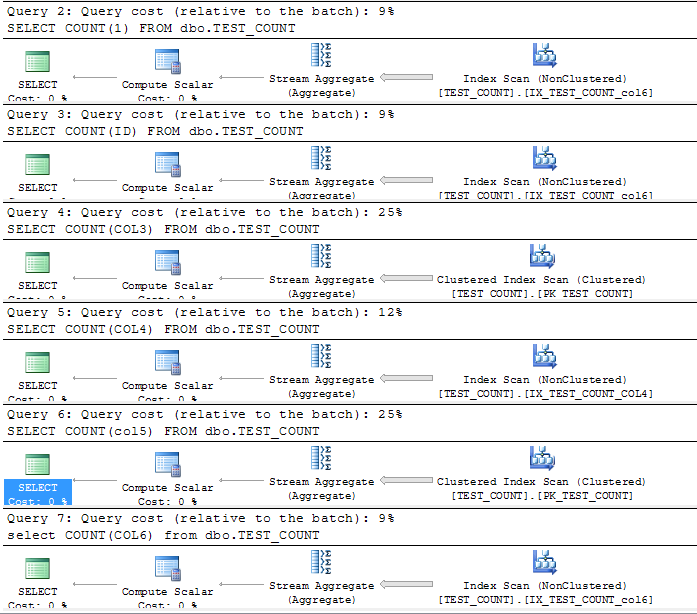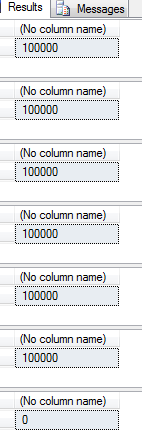select count(1),count(*),count(主键)，那sqlserver会选择扫描一个列最窄的一般的非聚集索引，因为这些非聚集索引上存储了我们的主键列。
select（一般索引列），sqlserver会选择扫描自己的索引.
select（筛选索引列）因为筛选索引的数据不全，而其他索引上又不包含该列信息，所以sqlserver选择扫描聚集索引。
select（一般数据列）因为没有哪个索引包含该列信息，所以sqlserver也选择扫描聚集索引

alter  table  DBO.TEST_COUNT
drop  constraint PK_TEST_COUNT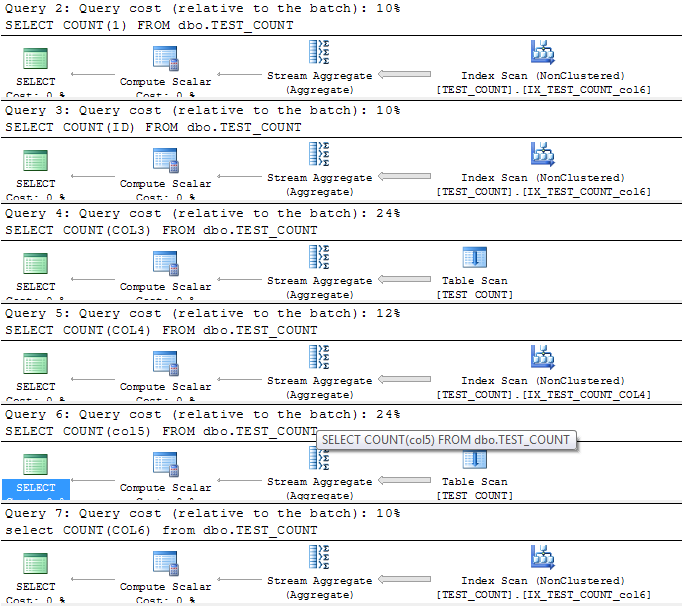select count(1),count(*),count(主键)，那sqlserver会选择扫描一个列最窄的一般的非聚集索引，因为这些非聚集索引上存储了我们的RID。
select（一般索引列），sqlserver会选择扫描自己的索引.
select（筛选索引列）因为筛选索引的数据不全，而其他索引上又不包含该列信息，所以sqlserver选择全表扫描。
select（一般数据列）因为没有哪个索引包含该列信息，所以sqlserver也选择全表扫描

• 博文量
28
• 访问量
69257